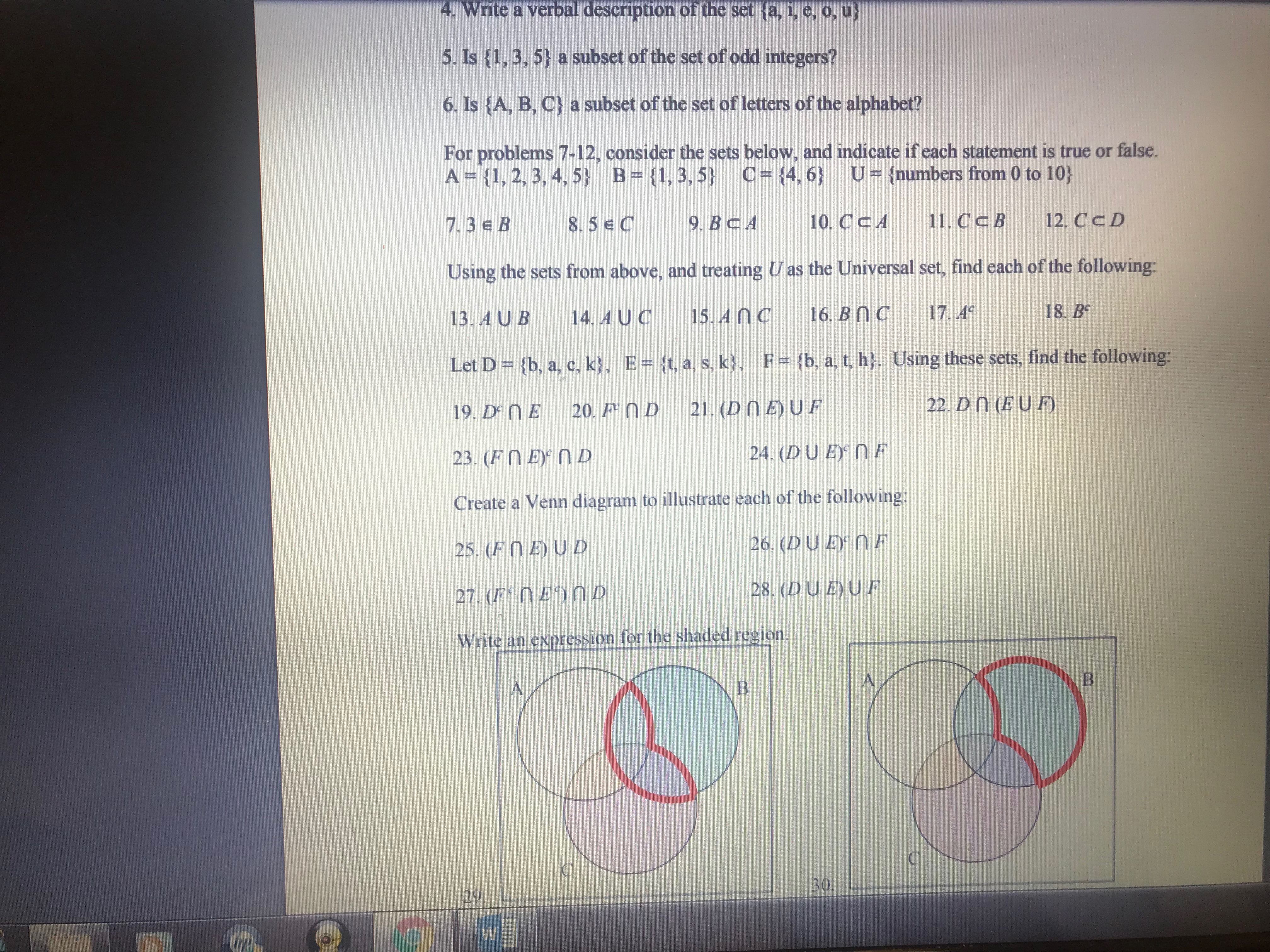# 4.Writeaverbaldescriptionofthesetta,i,e,o,u5. Is (1,3, 5) a subset of the set of odd integers?6. 1s {A, B, C} a subset of the set of letters of the alphabet?For problems 7-12, consider the sets below, and indicate if each statement is true or false.A 11, 2, 3, 4,5) B ,3,5 C14,6) Ufnumbers from 0 to 10)7.3e B 8.59. BcA10. CCA 1. CcB 12. CcDUsing the sets from above, and treating U as the Universal set, find each of the following:13. A UB 14. AUC 15.AnC 16.BnC 17.e 18. BeLet D- (b, a, c, k, E- It, a, s, k), F (b, a, t, h). Using these sets, find the following:19. D NE 20. D 21. (DnE)UF23. (FnE n DCreate a Venn diagram to illustrate each of the following:25. (F EJUD22. Dn (EUF)24. (DUE NF26. (DUE)NF28. (DUEUFWrite an expression for the shaded region.3029

Question
52 viewshelp_outlineImage Transcriptionclose4. Write a verbal description of the set ta, i, e, o, u 5. Is (1,3, 5) a subset of the set of odd integers? 6. 1s {A, B, C} a subset of the set of letters of the alphabet? For problems 7-12, consider the sets below, and indicate if each statement is true or false. A 11, 2, 3, 4,5) B ,3,5 C14,6) Ufnumbers from 0 to 10) 7.3e B 8.59. BcA10. CCA 1. CcB 12. CcD Using the sets from above, and treating U as the Universal set, find each of the following: 13. A UB 14. AUC 15.AnC 16.BnC 17.e 18. Be Let D- (b, a, c, k, E- It, a, s, k), F (b, a, t, h). Using these sets, find the following: 19. D NE 20. D 21. (DnE)UF 23. (FnE n D Create a Venn diagram to illustrate each of the following: 25. (F EJUD 22. Dn (EUF) 24. (DUE NF 26. (DUE)NF 28. (DUEUF Write an expression for the shaded region. 30 29 fullscreen
check_circle

Step 1

14) A U C :

Step 2

16) B ^ C...

### Want to see the full answer?

See Solution

#### Want to see this answer and more?

Solutions are written by subject experts who are available 24/7. Questions are typically answered within 1 hour.*

See Solution
*Response times may vary by subject and question.
Tagged in

### Applications of Mathematics